EngliSea > M > math > 25 Exponent, root and logarithm

+plus (−minus) ↺ ·times (/over) ↺ ˄to (˅root ⍻log)

Expansion of Numbers and Operations
+addition (−subtraction) ↺ ·multiplication (/division) ↺ ˄exponentiation (˅root ⍻logarithm)
4882

Exponentiation Properties
① (p˄m)·(p˄n) = p˄(m+n) ② (p˄m)˄n = p˄(m·n) ③ (p·q)˄n = (p˄n)·(q˄n)
5283

Logarithm Properties
① 1⍻b = 0 ② b⍻b = 1 ③ (b˄x)⍻b = x ④ b˄(x⍻b) = x ⑤ (x˄p)⍻b = p·(x⍻b) ⑥ x⍻b = (x⍻c)/(b⍻c) ⑦ (x·y)⍻b = x⍻b+y⍻b ⑧ (x/y)⍻b = x⍻b-y⍻b
966605 Exponents In Algebra
Algebra Basics: Exponents In Algebra - Math Antics
3799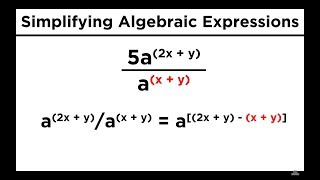08 Simplifying Expressions With Roots and Exponents
Simplifying Expressions With Roots and Exponents
3801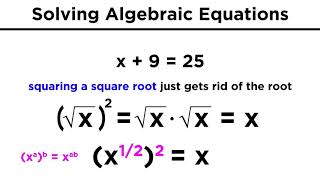09 Solving Algebraic Equations With Roots and Exponents
Solving Algebraic Equations With Roots and Exponents
3802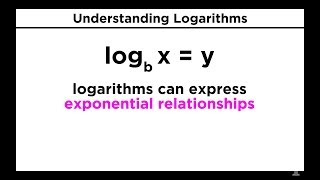41 Logarithms Part 1
When we say log base b of x equals y, we are saying b to the y equals x. With logs, the base of the log raised to the power of what's on the other side of the equal sign will equal the number that the log is operating on.
3638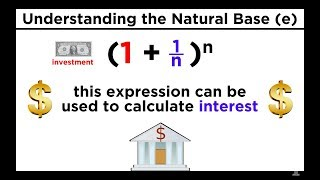42 Logarithms Part 2
Base Ten Logs, Natural Logs, and the Change-Of-Base Property
3639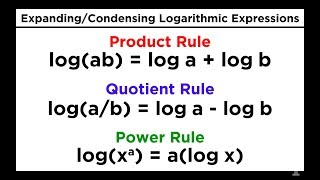43 Logarithms Part 3
Logarithms Part 3: Properties of Logs, Expanding Logarithmic Expressions
3640Change of base
Log base b of n. What power should I put in the exponent of b so b to that power will give me n.
3798exponential function
You can expect the difference between one x value at any point to be the same as any other.
3625◌◌◌ Exponentiation
b˄n is called "b raised to the n-th power", "b raised to the power of n", "the n-th power of b", "b to the n-th", or most briefly as "b to the n"
9671

◌◌◌ Interest
Simple And Compound Interest And Spreadsheet Intro
9774◌◌◌ Logarithm
"the log, base b, of x" "To what power must b be raised, in order to yield x?"
9638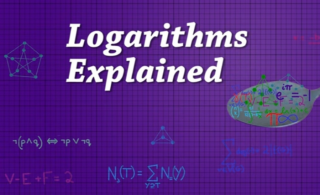Logarithms Explained
In the same way that division is the inverse of multiplication a logarithm is just the inverse of exponentiation.
3738

Logarithms Explained
What is the power that I should raise this base to in order to get this number?
9635

Logarithms Explained
Anytime you see log base b of some number n, you can think about it as asking the question "What power do I need to put in my exponent to get b to that power equal to this number n?"
9637

x⍻b
ᐥWhat power do you have to raise b to in order to get x?ᐥ
17402-